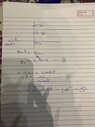# Independence of vertical and horizontal motion

• B
• rudransh verma
In summary, the equation of motion for the falling mass is pretty easy, right? and the equation of motion for the projectile will have a horizontal and a vertical component.f
@Doc Al Now what?
Again, it's a bit difficult to understand what you've written. Please type out your equations here, not handwritten on paper.

(A) Start here: In terms of the horizontal distance traveled (I'd call it "d") and the angle of the ball's initial velocity (θ) express the initial height of the can.

(B) Solve for the travel time for the shot to cover that horizontal distance. (In terms of those variables.)

(C) Write an expression for the height of the ball at that time. (Measured from ground level.)

(D) Write an expression for the height of the can at that time. (Measured from ground level -- that's where the initial height of the can is needed.)

Compare!

Last edited:
D) Write an expression for the height of the can at that time. (Measured from ground level -- that's where the initial height of the can is needed.)
I have written 3. I don’t understand how to write 4th
@berkeman help!

#### Attachments

•image.jpg
39.9 KB · Views: 46
Last edited:
I have written 3. I don’t understand how to write 4th
@berkeman help!
So far, so good. For (C) you wrote the height as a function of time -- now plug in the time, which you found in step (B).

For (D), use the same approach but realize that you aren't starting from y = 0, but y = y2 (which you found in step (A)).

For (D), use the most general form of the kinematic equation:
y = y0 + v0,y t + (1/2) a t2

You know y0; you found that in (A). You know the initial velocity of the can. And you know the acceleration due to gravity.

For (D), use the same approach but realize that you aren't starting from y = 0, but y = y2 (which you found in step (A)).
y3 in first box is vertical distance covered by ball whereas y3 in second box is the height of can after time t. For first y3 I took starting point as 0. For second y3 I took starting point as y2 and applied y3-y2= -1/2gt^2
But is it right to take final point as y3 and initial as y2 because y2>y3. Isn’t this negative displacement?

#### Attachments

For second y3 I took starting point as y2 and applied y3-y2= -1/2gt^2
Good. Rearrange this to give the position of the can at time t: y3 = y2 -1/2gt^2

But is it right to take final point as y3 and initial as y2 because y2>y3. Isn’t this negative displacement?
The can is falling, after all. So its displacement from the starting point is negative. So what?

Now find its position at the time you calculated in step (B). And compare that position to that of the ball, which you should have done for step (C).

Now find its position at the time you calculated in step (B). And compare that position to that of the ball, which you should have done for step (C).
Already done it. It’s shown in image above in the boxes. Both y3s at time t are same.
So at the same time t both the y3 are same. This means position of the can and ball are same after time t from below and above. If both y3 had different values they would not have hit each other.

Excellent.

•rudransh verma
Excellent.
So y2 and origin are fixed but y3 is not. To make it more clear I could have taken y31 and y32.
Thank you
But I have a question. We took the initial velocity of can to be zero. Why have we taken initial velocity of ball not zero?

Why have we taken initial velocity of ball not zero?
The ball is being shot at the can. If its initial velocity were zero, it would just sit there. (It's already on the ground.) On the other hand, the can is being dropped from rest.

The ball is being shot at the can. If its initial velocity were zero, it would just sit there. (It's already on the ground.) On the other hand, the can is being dropped from rest.
So when we do something against gravity we take initial velocity as nonzero of the body but when we leave body in gravity we say it started from rest?

So when we do something against gravity we take initial velocity as nonzero of the body but when we leave body in gravity we say it started from rest?
It all depends on the problem you're trying to analyze. In this problem, we are shooting a projectile at a target that is dropped from rest.